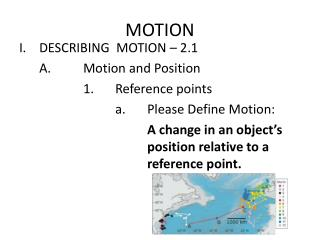# MOTION - PowerPoint PPT PresentationDownload PresentationMOTION

MOTIONDownload Presentation## MOTION

- - - - - - - - - - - - - - - - - - - - - - - - - - - E N D - - - - - - - - - - - - - - - - - - - - - - - - - - -
##### Presentation Transcript

1. MOTION • DESCRIBING MOTION – 2.1 A. Motion and Position 1. Reference points a. Please Define Motion: A change in an object’s position relative to a reference point.

2. Please Define Reference Point: An arbitrary position selected for an object to start its movement toward a certain direction. • Please Define Frame of Reference: Is a coordinate system in which the position of the object is measured.

3. 2. Coordinate systems • A coordinate system is like a map, and the Reference Point is at the origin. • Change in Position 1. Distance a. Please Define Distance: The length an object travels, measured in SI units of meters. Distance is scalar quantity having magnitude but no direction.

4. 2. Displacement • Please Define Displacement: Distance and direction of an object’s change in position from the starting point. Displacement is a vector quantity having magnitude (size) and direction. 3. Adding displacements

5. C. Speed • Please Define Speed: Distance an object travels per unit of time. Speed is a scalar quantity having magnitude (size) but no direction. • Calculating speed a. What is the equation for speed? Speed = distance (in meters) time (in seconds) S = d t

6. 3. Practice Problems p.47 1. 2. 3. 4.

7. 4. Constant speed • Please Define Constant Speed: An object neither slows down nor speeds up, the car is traveling at a constant speed. The graph of an object traveling at constant speed is a straight line. • Changing speed a. Please Define Changing Speed: Speed is not constant but changes, i.e. speed increases, decreases, or the object stops.

8. 6. Average speed • Please Define Average Speed: The total distance an object travels divided by the total time it takes to travel that distance. • Instantaneous speed a. Please Define Instantaneous speed: Speed of an object at a given point in time.

9. D. Graphing Motion • Distance Time Graph • Speed on distance-time graphs a. Constant speed equals constant slope or is a straight line. b. Greater Speed equals steeper slope. c. At Rest equals no slope (a plateau).

10. II. VELOCITY AND MOMENTUM -2.1 A. Velocity 1. Please Define Velocity: The speed and direction of a moving object. Velocity unlike speed is a vector quantity having magnitude (size) and direction. 2. Velocity and speed a. Same speed, different velocities Two objects can have the same speed but different velocities because each object is moving in a different direction.

11. Motion of Earth’s Crust • Relative Motion • Momentum 1. Please Define Momentum: Property of a moving object that equals its mass times its velocity. 2. What is the Equation for Momentum? Momentum = mass x velocity p = m x v 3. Write the units for Momentum: p = kg m/s

12. 4. Comparing momentums • Two objects have the same mass but one object has a greater velocity, therefore that object has the greater momentum. b. Two objects have different masses and travel at the same speed, however, the heavier massed object would have the greater momentum.

13. III. ACCELERATION – 2.3 • Velocity and Acceleration 1. Please Define Acceleration: Rate of change of velocity; can be calculated by dividing the change in the velocity by the time it takes the change to occur. a. Acceleration is a vector quantity. Meaning acceleration has magnitude (size) and direction.

14. b. Write the equation for acceleration: Acceleration = change in velocity (m/s) m time ( in seconds) s2 • Speed-time graphs and acceleration • Calculating acceleration a. Write the Acceleration Equation:

15. b. Practice Problems p. 58 22. 23. 24.

16. B. Motion in Two Dimensions • Circular motion a. Why is an object moving in a circular path even if its speed remains constant, the object is accelerating? The object is accelerating because the direction of the motion of that object constantly changes.

17. b. Please Define Centripetal Acceleration: Acceleration of an object toward the center of a curved or circular path.

18. 2. Projectile motion • Horizontal and vertical motion • Throwing and dropping 1. Which ball(s) have the same vertical acceleration? Both Red and Blue balls 2. Which ball(s) have the same horizontal displacement? Red ball only • Which ball(s) reach the ground at the same time? Both Red and Blue balls • Which ball(s) have no horizontal velocity? Blue ball • Which ball(s) have only vertical acceleration? Red ball only? Blue ball only

19. 2. Projectile motion

20. 3. Amusement park acceleration